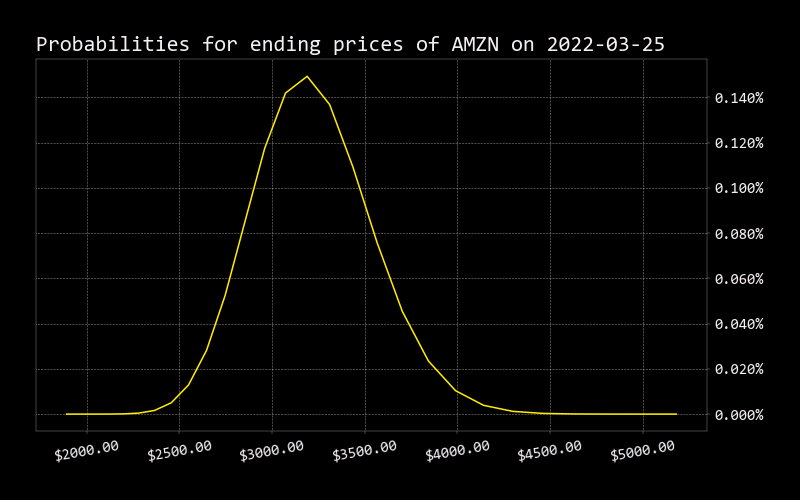# Binom

``````usage: binom [-s STRIKE] [-p] [-e] [-x] [--plot] [-v VOLATILITY] [-h]
``````

Shows the value of an option using binomial option pricing. Can also show raw data and provide a graph with predicted underlying asset ending values. The binomial options model calculates how big an up step or down step in the next time period will likely be. Then it creates a tree doing this at each period. The end results of this is a tree with possible asset values at each “step”. For our calculations we use a day as our “step” time period. We then take all of the expected values at the finishing date and use this to begin a tree of option values at each step. The ending results is the value of the option today.

The up step is calculated by taking e to the power of volatility times the square root of the change in time during the step. This is the percentage we expect the stock to increase if there is an upward movement. The down step is the inverse of the up step. The probability of the up step is calculated by taking e to the power of the risk free rate minus the dividend yield. This is multiplied by the change in time for the step and then subtracted by the expected downward movement. This number is then divided by the up step subtracted by then down step. The probability of a downward step is just one minus the probability of an upward step.

Formulas: up_step = e ^ (volatility * (delta_t ^ (1 / 2))) down_step = 1 / up_step

prob_up = (e ^ ((risk_free - div_yield) * delta_t) - down_step) / (up_step - down_step) prob_down = 1 - prob_up

``````optional arguments:
-s STRIKE, --strike STRIKE
Strike price for option shown (default: 0)
-p, --put             Value a put instead of a call (default: False)
-e, --european        Value a European option instead of an American one (default: False)
-x, --xlsx            Export an excel spreadsheet with binomial pricing data (default: False)
--plot                Plot expected ending values (default: False)
-v VOLATILITY, --volatility VOLATILITY
Underlying asset annualized volatility. (None indicates that the historical volatility is being used) (default: None)
-h, --help            show this help message (default: False)
``````

Example:

``````2022 Feb 16, 08:40 (✨) /stocks/options/ \$ binom -s 3100 -e --plot

AMZN call at \$3100.00 expiring on 2022-03-25 is worth \$136.85

2022 Feb 16, 08:41 (✨) /stocks/options/ \$ binom -s 3500 -p --plot

AMZN put at \$3500.00 expiring on 2022-03-25 is worth \$389.72
``````